# Solving Quadratic Systems of Equations via Truncated Wirtinger Flow

For random designs: computational cost of TWF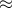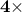that of solving Least Squares of the same size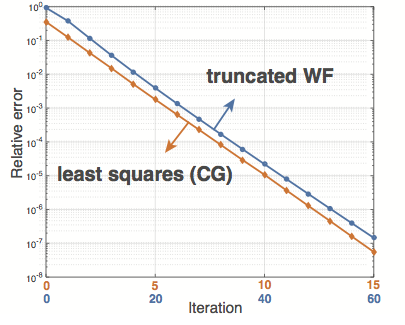Truncated Wirtinger Flow (TWF) is a non-convex procedure for solving quadratic equations: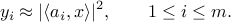the data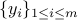and design vectors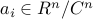are known;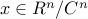is unknown.

Solving Random Quadratic Systems of Equations Is Nearly as Easy as Solving Linear Systems. Y. Chen and E. J. Candès.

We consider the fundamental problem of solving quadratic systems of equations in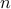variables, where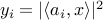,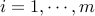and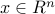is unknown. We propose a novel method, which starting with an initial guess computed by means of a spectral method, proceeds by minimizing a nonconvex functional as in the Wirtinger flow approach. There are several key distinguishing features, most notably, a distinct objective functional and novel update rules, which operate in an adaptive fashion and drop terms bearing too much influence on the search direction. These careful selection rules provide a tighter initial guess, better descent directions, and thus enhanced practical performance. On the theoretical side, we prove that for certain unstructured models of quadratic systems, our algorithms return the correct solution in linear time, i.e. in time proportional to reading the data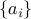and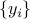as soon as the ratio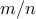between the number of equations and unknowns exceeds a fixed numerical constant. We extend the theory to deal with noisy systems in which we only have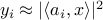and prove that our algorithms achieve a statistical accuracy, which is nearly un-improvable. We complement our theoretical study with numerical examples showing that solving random quadratic systems is both computationally and statistically not much harder than solving linear systems of the same size — hence the title of this paper. For instance, we demonstrate empirically that the computational cost of our algorithm is about four times that of solving a least-squares problem of the same size.